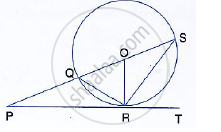Share

# In the Given Figure, Pt Touches the Circle with Centre O at Point R. Diameter Sq is Produced to Meet the Tangent Tr at P. Given ∠Spr = X° and ∠Qrp = Y°; (Ii) Write an Expression Connecting X and Y. - Mathematics

Course

#### Question

In the given figure, PT touches the circle with centre O at point R. Diameter SQ is produced to meet the tangent TR at P.
Given ∠SPR = x° and ∠QRP = y°;
Prove that:
(i) ∠ORS = y°
(ii) Write an expression connecting x and y.#### Solution

∠QRP = ∠OSR = y (angles in alternate segment)

But OS = OR (Radii of the same circle)
∴∠ORS = ∠OSR = y
∴ OQ = OR (radii of same circle)
∴ OQR = ∠ ORQ=  90° - y …………(i) (Since OR ⊥ PT )
But in Δ PQR ,
Ext ∠ OQR = x  + y ……(i)
From (i) and (ii)
x + y  = 90°- y
⇒ x  + 2 y = 90°

Is there an error in this question or solution?